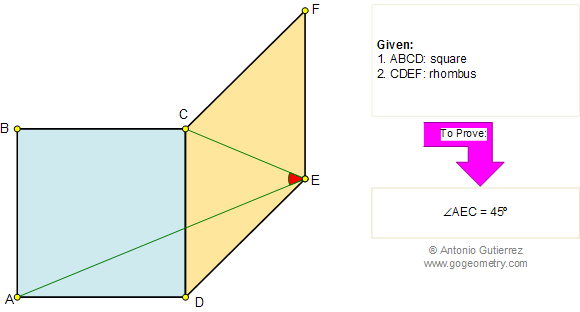Problem 179. Rhombus, Square, Diagonal, Angle.
 In the figure below, ABCD is a square and CDEF is a rhombus. Prove that angle AEC is a 45 degree angle. A rhombus or rhomb is an equilateral quadrilateral. Notice that it is a parallelogram. View or post a solution.Home | Geometry | Search | Problems | 171-180 | Email | By Antonio Gutierrez